﻿ 全直流供电建筑储能最优运行策略研究

# 全直流供电建筑储能最优运行策略研究Research on Optimal Operation Strategy of All-DC Power Supply for the Building Energy Storage

Abstract: Compared with traditional AC power supply buildings, the All-DC power supply buildings have great advantages in terms of safety, economy and power quality optimization. Studying the optimal operation strategy of the All-DC building’s energy storage, the main purpose is to coordinate the charging and discharging of the internal energy storage of the building through the construction of the All-DC power distribution/electricity, and the peak-to-valley difference caused by the fluctuation of urban power load daily electricity consumption could be fully adjusted and optimized. Based on the above concepts, a new type of the All-DC power supply building with a variety of new energy equipment, time-of-day power generation, time-consuming energy consumption, etc., simulation, acquisition of power generation, energy storage and power consumption curves of a variety of equipment, establish power supply mathematics model. The operation strategy is optimized for the power supply of the constant power grid, the optimal power price and the demand side power take-off, and the operation strategy is obtained.

1. 引言

2. 全直流建筑供电模型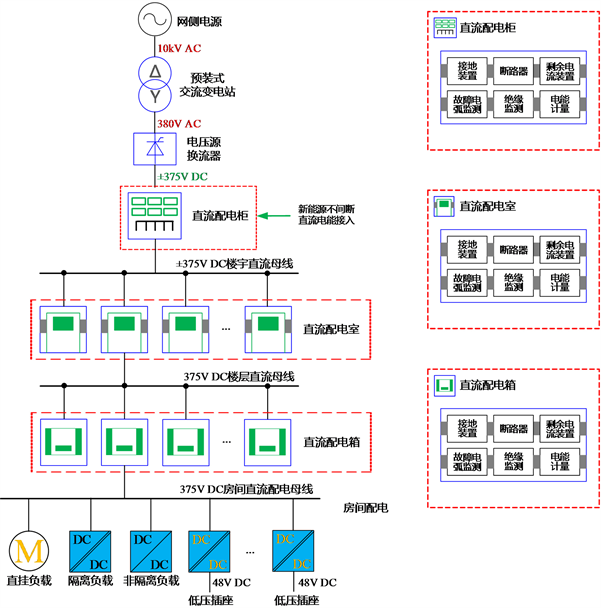Figure 1. Zero-carbon research office building full DC power supply system topology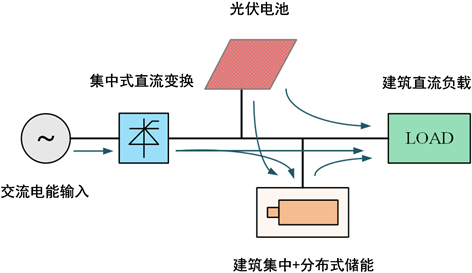Figure 2. Zero-carbon research office building power supply model

1) 电网取电：可取功率为DC2 × 200 kW；

2) 光伏发电：最大取电功率约100 kWp；

1) 集中式储能，布置于集中储能柜内，约100 kWh；

2) 分布式储能：分别布置于空调、房间等空间内，有效储能量约30 kWh；

3. 优化目标与优化方式

$W=\left[{w}_{1},{w}_{2},\cdots ,{w}_{24}\right]$ (1)

$V=\left[{v}_{1},{v}_{2},\cdots ,{v}_{24}\right]$ (2)

$B=\left[{b}_{1},{b}_{2},\cdots ,{b}_{24}\right]$ (3)

$P=\left[{p}_{1},{p}_{2},\cdots ,{p}_{24}\right]$ (4)

$P=W-V-B$ (5)

$\left\{\begin{array}{l}{\sum }_{i=1}^{24}{b}_{i}=0\\ 0\le {b}_{j}\le Q\\ SOC=\frac{Q-{\sum }_{i=1}^{j}{b}_{i}}{Q}×100\\ SOC\in \left(0,100\right]\end{array}$ (6)

${F}_{1}\left(P\right)={\sum }_{i=1}^{24}{\left({p}_{i}-\stackrel{¯}{p}\right)}^{2}$ (7)

$\stackrel{¯}{p}=\frac{{\sum }_{i=1}^{24}{p}_{i}}{24}$ (8)

$\mathrm{min}\text{\hspace{0.17em}}{F}_{1}\left(P\right)={\sum }_{i=1}^{24}{\left({p}_{i}-\stackrel{¯}{p}\right)}^{2}$ (9)

$\mathrm{min}\text{\hspace{0.17em}}{F}_{2}\left(P\right)={\sum }_{i=1}^{23}\left({p}_{i}-{p}_{i-1}\right)$ (10)

$M=\left[{m}_{1},{m}_{2},\cdots ,{m}_{24}\right]$ (11)

$\mathrm{min}\text{\hspace{0.17em}}{F}_{3}\left(P\right)={\sum }_{i=1}^{24}{p}_{i}×{m}_{i}$ (12)

$G=\left[{g}_{1},{g}_{2},\cdots ,{g}_{24}\right]$ (13)

$\mathrm{min}\text{\hspace{0.17em}}{F}_{4}\left(P\right)={\sum }_{i=1}^{24}|{p}_{i}-{g}_{i}|$ (14)

4. 储能优化策略与控制对象

${p}_{set}=\stackrel{¯}{p}$ (15)

2) 储能电池容量≥充放电周期内超出均值能量的累积值:

$Q\ge {\sum }_{i=1}^{24}\left({p}_{i}^{+}-\stackrel{¯}{p}\right)$ (16)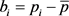(17)

1) 比例充放，即以小时耗电量与小时耗电量成比例补偿：

${b}_{k}=\frac{2Q\left({p}_{k}-\stackrel{¯}{p}\right)}{{\sum }_{j=1}^{24}|\stackrel{¯}{p}-{p}_{j}|}$ (18)

2) 平均充放，即每小时放电/充电量相同进行补偿：

$\left\{\begin{array}{l}{\stackrel{¯}{b}}^{+}=\frac{Q}{length\left({p}_{k}>\stackrel{¯}{p}\right)}\\ {\stackrel{¯}{b}}^{-}=\frac{Q}{length\left({p}_{k}<\stackrel{¯}{p}\right)}\end{array}$ (19)

3) 峰值充放，即只在用电峰值3小时与用电谷值5小时进行放电/充电补偿，其余时间储能电池不充不放。

5. 优化仿真Table 1. Modeling and simulation parameters

$\begin{array}{l}{P}_{0冬}={W}_{冬}-{V}_{冬}=\left[37.85,75.21,55.05,45.88,48.58,56.14,64.58,47.19,57.38,69.70,48.93,\\ \text{ }\text{ }\text{ }\text{ }\text{ }\text{ }\text{\hspace{0.17em}}\text{\hspace{0.17em}}\text{\hspace{0.17em}}\text{\hspace{0.17em}}26.87,25.33,22.62,19.33,8.55,6.70,3.03,3.03,3.03,3.03,3.03,3.03,15.40\right]\\ {P}_{0夏}={W}_{夏}-{V}_{夏}=\left[66.83,70.33,113.40,98.48,78.62,74.79,67.06,69.79,61.02,73.75,93.94,\\ \text{ }\text{ }\text{ }\text{ }\text{ }\text{ }\text{\hspace{0.17em}}\text{\hspace{0.17em}}\text{\hspace{0.17em}}\text{\hspace{0.17em}}78.45,42.11,46.36,45.73,44.61,8.64,6.78,3.12,3.12,3.12,3.12,3.12,2.49\right]\end{array}$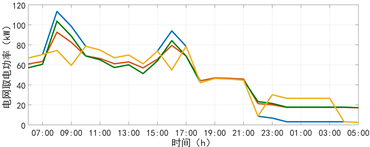a冬季电网取电优化曲线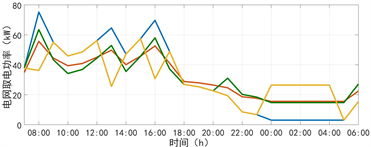b夏季电网取电优化曲线

Figure 3. 3 kinds of energy storage and charging optimization strategies optimization curve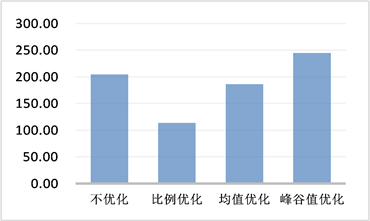(a) 冬季评判值方差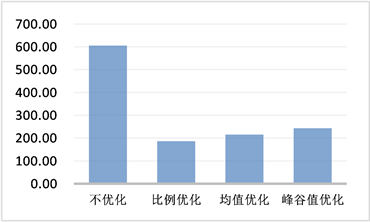(b) 冬季评判值平滑度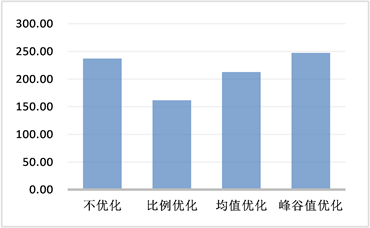(c) 夏季评判值方差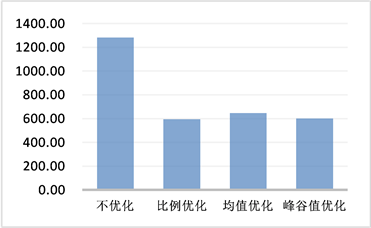(d) 夏季评判值平滑度

Figure 4. Comparison of evaluation values of 3 kinds of energy storage and charging optimization strategies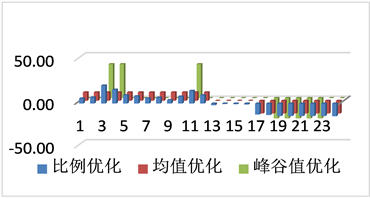(a) 冬季储能24小时充/放电能量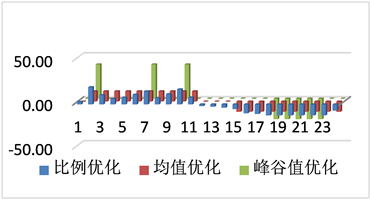(b) 夏季储能24小时充/放电能量

Figure 5. Winter/Summer 24 hour charge/discharge energy values under 3 optimization strategies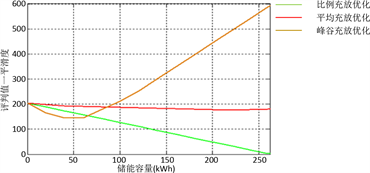Figure 6. Evaluation values of three optimization strategies and energy storageTable 2. 3 kinds of energy storage and charging optimization strategy evaluation value

6. 结论

 张风燕, 郭红斌, 纪承承, 等. 一种新型光伏节能直流建筑[P]. CN205134669U, 2016.

 程兆祺, 李奥, 王福林. 建筑直流供电和分布式蓄电系统的仿真研究[J]. 建筑电气, 2017, 36(7): 18-22.

 王福林, 江亿. 建筑全直流供电和分布式蓄电关键技术及效益分析[J]. 建筑电气, 2016, 35(4): 16-20.

 马化盛. 解析直流智慧照明在节能建筑中的应用[C]//中国照明论坛——半导体照明创新应用与智慧照明发展论坛. 2017.

 阎吉. 低压直流供电系统研究综述[J]. 建筑工程技术与设计, 2016(25): 1063, 1071.

 丁宝, 张进, 赵亮, 等. 建筑直流配电系统研究[J]. 智能建筑电气技术, 2013(6): 9-12.

 黄冬艳, 陆佩芳. 可持续节能建筑的直流供电架构[J]. 智能建筑电气技术, 2013(3): 81-85.

 Sedghi, M., Ahmadian, A. and Aliak-bar-Golkar, M. (2016) Optimal Storage Planning in Active Distribution Network Considering Uncertainty of Wind Power Distributed Generation. IEEE Transactions on Power Systems, 31, 304-316.
https://doi.org/10.1109/TPWRS.2015.2404533

 Nick, M., Cherkaoui, R. and Paolone, M. (2014) Optimal Allocation of Dispersed Energy Storage Systems in Active Distribution Networks for Energy Balance and Grid Support. IEEE Transactions on Power Systems, 29, 2300-2310.
https://doi.org/10.1109/TPWRS.2014.2302020

 杨秀, 陈洁, 朱兰, 等. 基于经济调度的微网储能优化配置[J]. 电力系统保护与控制, 2013(1): 53-60.

 许健, 刘念, 于雷, 等. 计及重要负荷的工业光伏微电网储能优化配置[J]. 电力系统保护与控制, 2016, 44(9): 29-37.

 王伟, 薛金花, 叶季蕾, 等. 基于SOC调控的用于抑制光伏波动的电池储能优化控制方法[J]. 电力系统保护与控制, 2014(2): 75-80.

 白牧可, 唐巍, 谭煌, 等. 基于虚拟分区调度和二层规划的城市配电网光伏–储能优化配置[J]. 电力自动化设备, 2016, 36(5): 141-148.

 张丹丹, 童亦斌, 金新民, 等. 计及需求响应的微电网储能优化配置[J]. 电力电子技术, 2016(11): 107-109.

 孟秋艳, 童亦斌, 梁建钢. 基于电池单体电压和SOC的储能优化控制策略[J]. 电气传动, 2018(5): 63-67.

 赵晶晶, 徐传琳, 吕雪, 等. 微电网一次调频备用容量与储能优化配置方法[J]. 中国电机工程学报, 2017(15): 4324-4332.

Top